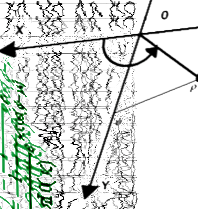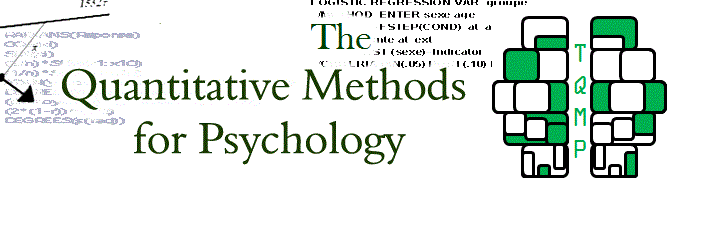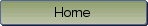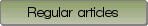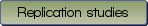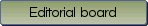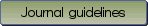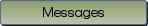How to use MATLAB to fit the ex-Gaussian and other probability functions to a distribution of response times

Bibliographic information: BibTEX format RIS format XML format APA style
Cited references information: BibTEX format APA style

Lacouture, Yves , Cousineau, Denis
35-45
Keywords: Quantitative methods , Distribution fitting
Tools: Matlab
(no sample data)   (Appendix)

This article discusses how to characterize response time (RT) frequency distributions in terms of probability functions and how to implement the necessary analysis tools using MATLAB. The first part of the paper discusses the general principles of maximum likelihood estimation. A detailed implementation that allows fitting the popular ex-Gaussian function is then presented followed by the results of a Monte Carlo study that shows the validity of the proposed approach. Although the main focus is the ex-Gaussian function, the general procedure described here can be used to estimate best fitting parameters of various probability functions. The proposed computational tools, written in MATLAB source code, are available through the Internet.

Be informed of the upcoming issues with RSS feed:RSS